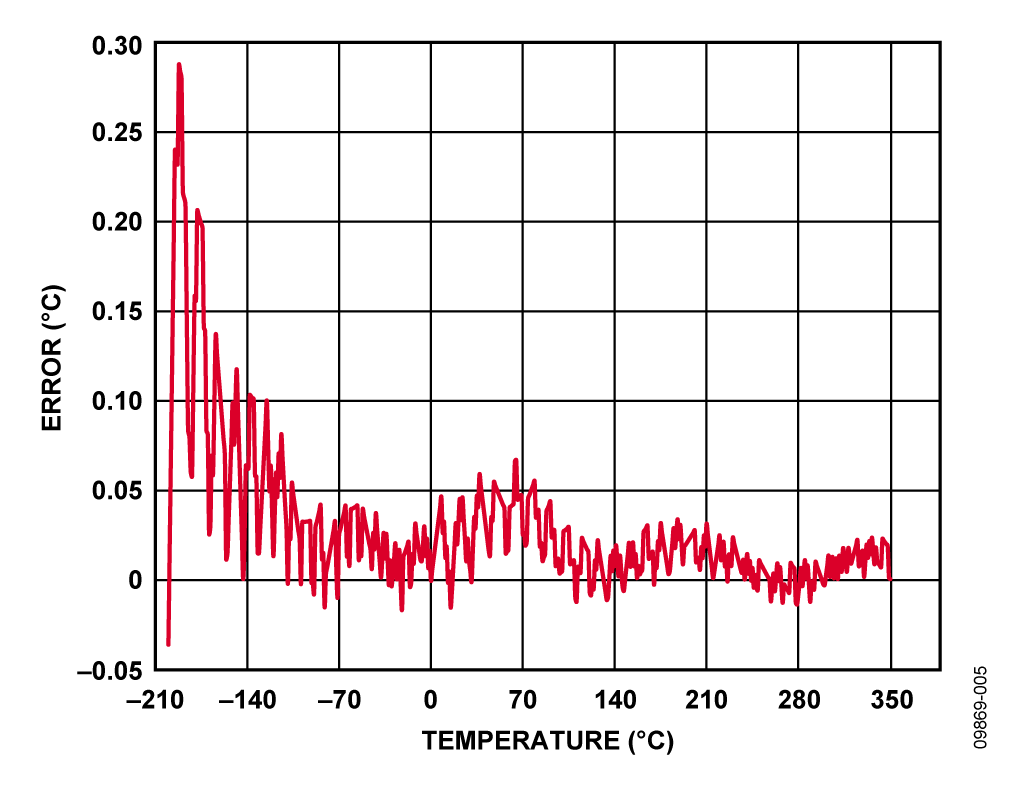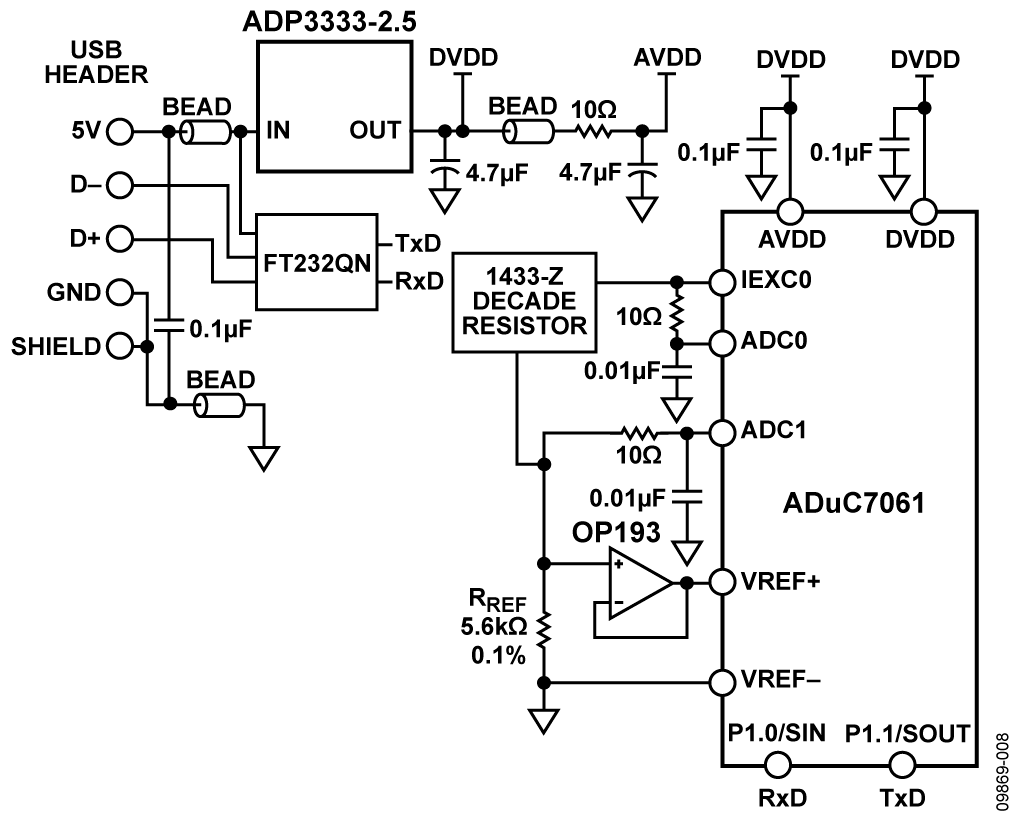Оценочные платы \$25.75## Ресурсы проектирования

### Файлы проектирования и интеграции

• Schematic
• Bill of Materials
• Gerber Files
• Assembly Drawing
Загрузить файлы проектов 477 kB

### Оценочные платы

Буква "Z" в наименовании компонента указывает на соответствие требованиям RoHS. Отмеченные платы нужны для оценки данной схемы

• EVAL-ADUC7061MKZ (\$25.75) ADUC7061 evaluation board used to evaluate this circuit. Please see "Circuit Evaluation & Test" section for configuration information.

## Особенности и преимущества

• Typical temperature range of -200 C to +400 C
• T-type thermocouple measurement system
• Single chip solution with an ARM 7 processing core
• Cold junction compensation using an RTD

## Функции и преимущества схемы

This circuit uses the ADuC7060/ADuC7061 precision analog microcontroller in an accurate thermocouple temperature monitoring application. The ADuC7060/ADuC7061 integrates dual 24-bit Σ-Δ ADCs, dual programmable current sources, a 14-bit DAC, and a 1.2 V internal reference, as well as an ARM7 core, 32 kB flash, 4 kB SRAM, and various digital peripherals such as UART, timers, SPI, and I2C interfaces.

In the circuit, the ADuC7060/ADuC7061 are connected to a thermocouple and a 100 Ω Pt RTD. The RTD is used for cold junction compensation.

In the source code, an ADC sampling rate of 4 Hz is chosen. When the ADC input PGA is configured for a gain of 32, the noise free code resolution of the ADuC7060/ADuC7061 is greater than 18 bits.Figure 1. ADuC7060/ADuC7061 As a Temperature Monitor Controller with a Thermocouple Interface (Simplified Schematic, All Connections Not Shown)

## Описание схемы

Application Note AN-0970, RTD Interfacing and Linearization Using an ADuC706x Microcontroller. The following features of the ADuC7060/ADuC7061 are used in this application:

• Primary 24-bit Σ-Δ ADC with programmable gain amplifier (PGA): The PGA is set for a gain of 32 in the software for this application. The primary ADC was continuously switched between sampling the thermocouple and the RTD voltages.

• Programmable excitation current sources for forcing a controlled current through the RTD: The dual current sources are configurable in 200 μA steps from 0 μA to 2 mA. For this example, a 200 μA setting was used to minimize the error introduced by the RTD self-heating.

• Internal 1.2 V reference for the ADC in the ADuC7060/ADuC7061: For measuring the thermocouple voltage the internal voltage reference was used due to its precision.

• External voltage reference for the ADC in the ADuC7060/ ADuC7061: For measuring the RTD resistance, we used a ratiometric setup where an external reference resistor (RREF) is connected across the external VREF+ and VREF− pins.

• 14-bit DAC: The DAC was used to set the thermocouple common-mode voltage to 850 mV above ground.

• ARM7TDMI® core: The powerful 16-/32-bit ARM7 core with integrated 32 kB flash and SRAM memory runs the user code that configures and controls the ADC, processes the ADC conversions from the RTD, and controls the communications over the UART/USB interface.

• UART: The UART was used as the communication interface to the host PC.

• Two external switches are used to force the part into its flash boot mode: By holding S1 low and toggling S2, the ADuC7060/ADuC7061 will enter boot mode instead of normal user mode. In boot mode, the internal flash may be reprogrammed through the UART interface.

Both the thermocouple and the RTD generate very small signals; therefore, a PGA is required to amplify those signals. As the auxiliary ADC on the ADuC7060/ADuC7061 does not have a PGA, both were connected to the primary ADC, and switching between the two was done in software.

The thermocouple used in this application is a type T (copperconstantan), which has a temperature range of −200°C to +350°C. Its sensitivity is about 40 μV/°C, which means that the ADC in the bipolar mode with a PGA gain of 32 can cover the entire temperature range of the thermocouple.

The RTD was used for cold junction compensation. The particular one used in this circuit was a platinum 100 Ω RTD, model number Enercorp PCS 1.1503.1. It is available in a 0805 surface-mount package. This RTD has a temperature variation of 0.385 Ω/°C.

Note that the reference resistor, RREF, should be a precision 5.62 kΩ (±0.1%).

The USB interface to the ADuC7060/ADuC7061 is implemented with an FT232R UART to USB transceiver, which converts USB signals directly to the UART.

In addition to the decoupling shown Figure 1, the USB cable itself should have a ferrite bead for added EMI/RFI protection. The ferrite beads used in the circuit were Taiyo Yuden, #BK2125HS102-T, which have an impedance of 1000 Ω at 100 MHz.

The circuit must be constructed on a multilayer PC board with a large area ground plane. Proper layout, grounding, and decoupling techniques must be used to achieve optimum performance (see Tutorial MT-031, Grounding Data Converters and Solving the Mystery of "AGND" and "DGND," Tutorial MT-101, Decoupling Techniques, and the ADuC7060/ ADuC7061 Evaluation Board layout).

The EVAL-ADUC7061MKZ PCB is shown in Figure 2.Figure 2. EVAL-ADUC7061MKZ Board Used for this Circuit.

Code Description

The source code used to test the circuit can be downloaded as a zip file from http://www.analog.com/CN0214-SourceCode.

The UART is configured for a baud rate of 9600, 8 data bits, no parity, no flow control. If the circuit is connected directly to a PC, a communication port viewing application such as HyperTerminal can be used to view the results sent by the program to the UART, as shown in Figure 3.

In order to get a temperature reading, the temperature of the thermocouple and of the RTD must be measured. The RTD temperature is converted to its equivalent thermocouple voltage via a lookup table. These two voltages are added together to give the absolute value at the thermocouple.

First, the voltage measured between the two wires of the thermocouple (V1). The RTD voltage is measured, converted to a temperature via a lookup table and this temperature is then converted to it’s equivalent thermocouple voltage (V2). V1 and V2 are then added to give the overall thermocouple voltage, and this is then converted to the final temperature measurement.

Initially, this was done using a simple linear assumption that the voltage on the thermocouple was 40 μV/°C. It can be seen from Figure 4. that this gives an acceptable error only for a small range around 0°C. A better way of calculating the thermocouple temperatures is to use a 6th order polynomial for the positive temperatures and a 7th order polynomial for the negative temperatures. This requires mathematical operations, which add to computational time and code size. A suitable compromise is to calculate the respective temperatures for a fixed number of voltages. These temperatures are stored in an array, and values in between are calculated using a linear interpolation between the adjacent points. It can be seen from Figure 5 that the error is drastically reduced using this method. Figure 5 gives the algorithm error using ideal thermocouple voltages. Figure 6 shows the error obtained when using ADC0 on the ADuC7060 to measure 52 thermocouple voltages over the full thermocouple operating range. The overall worst case error is <1°C.Figure 3. Output of HyperTerminal Communication Port Viewing ApplicationFigure 4. Error When Using Simple Linear ApproximationFigure 5. Error When Using Piecewise Linear Approximation Using 52 Calibration Points and ideal MeasurementsThe RTD temperature is calculated using lookup tables and is implemented for the RTD the same way as for the thermocouple.  Note that the RTD has a different polynomial describing its temperatures as a function of resistance.

For details on linearization and maximizing the performance of the RTD, refer to Application Note AN-0970, RTD Interfacing and Linearization Using an ADuC706x Microcontroller.

## Основные варианты исполнения

The ADP7102 regulator can be used as a newer alternate to the ADP3333. If more GPIO pins are required on the microcontroller, the ADuC7060, which comes in a 48-lead LFCSP or 48-lead LQFP package, is another option. Note that the ADuC7060/ADu7061 can be programmed or debugged via a standard JTAG interface.

When using the external reference resistor as the reference source for the RTD measurement, it is recommended to buffer the input to the VREF+ pin using an op amp in unity gain mode. This is to ensure the input leakage current to the VREF+ pin does not distort the measurement accuracy. In figure 8, we used an op amp, OP193 in unity gain for this purpose. This input is not buffered in the main diagram in Figure 1 but, for best results, a buffer is required.

For a standard UART to RS-232 interface, the FT232R transceiver could be replaced with a device such as the ADM3202, which requires a 3 V power supply. For a wider temperature range, a different thermocouple can be used, such as a type J. To minimize the cold junction compensation error, a thermistor could be placed in contact with the actual cold junction instead of on the PCB.

Instead of using the RTD and external reference resistor for measuring the cold junction temperature, an external digital temperature sensor could be used. For example, the ADT7410 could connect to the ADuC7060/ADuC7061 via the I2C interface.

For more details on cold junction compensation, please see Sensor Signal Conditioning, Analog Devices, Chapter 7, "Temperature Sensors."

If isolation between the USB connector and this circuit is required, the ADuM3160/ADuM4160 isolation devices should be added.

## Оценивание характеристик и тестирование схем

To test and evaluate the circuit, we evaluated the thermocouple measurements and the RTD measurements separately.

Thermocouple Measurement Test

The basic test setup is shown in Figure 6. Note that the thermocouple is connected to J2-8 and J2-9, and J2-5 must be connected to J2-8. The EVAL-ADUC7061MKZ board receives its power from the USB connection to the PC.

Two methods were used to evaluate the performance of the circuit. Initially the circuit was tested with the thermocouple attached to the board and used to measure the temperature of an ice bucket, then the temperature of boiling water.

A Wavetek 4808 Multifunction Calibrator was used to fully evaluate the error, as shown in Figure 4 and Figure 6. In this mode, the thermocouple was replaced with the calibrator as the voltage source, as shown in Figure 7. To evaluate the entire range of a T-type thermocouple, the calibrator was used to set the equivalent thermocouple voltage at 11 points between −200°C to +350°C for the negative and positive ranges of the T-type thermocouple. (See: ITS-90 Table for type T thermocouple).

To evaluate the accuracy of the lookup algorithm, 551 voltage readings, equivalent to temperatures in the range of −200°C to +350°C spaced at 1°C, were passed onto the temperature calculation functions. Errors were calculated for the linear method and the piecewise linear approximation method as can be seen in Figure 4 and Figure 5.Figure 7. Test Setup Used to Calibrate and Test the Circuit Over Full Thermocouple Output Voltage Range

RTD Measurement Test

To evaluate the RTD circuit and linearization source code, the RTD on the board was replaced with an accurate, adjustable resistance source. The instrument used was the 1433-Z Decade Resistor. RTD values from 90 Ω to 140 Ω which represents an RTD temperature range of -25C to 114C.

The setup circuit is shown in Figure 8.Figure 8. Test Setup Used to Calibrate and Test the RTD Output Voltage Range from –25°C to 114°C

The error results for the RTD tests are shown in Figure 9.Figure 9. Error in °C of RTD Measurement Using Piecewise Linearization Code and ADC0 Measurements